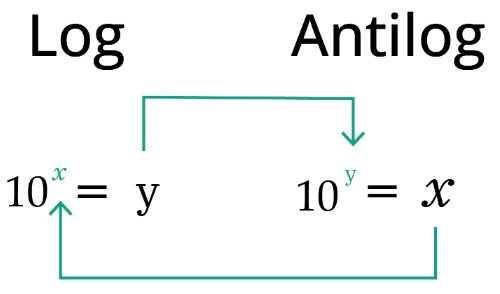# Antilog Calculator

Enter the number and base in antilog calculator to find the antilog.

Formula:
Antilog a (loga (x)) = x
Random Example

Give Us Feedback

## Antilog Calculator

This antilog calculator can undo the log process. It can find the value of antilog with just one click.

All you have to do is to enter the required values correctly in the designated boxes. Rest assured that the results are hundred percent accurate.

## How does antilog calculator work?

This inverse log calculator is very easy to use. You will need to;

1. Enter the number (log value).
2. Enter the antilog base.
3. Click “Calculate” or press enter.

## What is Antilog?

Anti means ‘Inverse’. It is the process of raising the log to its base. Since the log is the inverse of exponents, and antilog is the inverse of the log so eventually, antilog is equal to the exponent.In other words, it is the method of getting to the original value. For example:

Log (p) = q    ;    log-1(q) = p

Keep in mind that the base of antilog cannot be negative. Also, for a question where no base is provided, base 10 is used to find the antilog. It is because;

log(x) = log(10)

## Antilog formula

As explained before, the inverse logarithm is actually exponential. So it has the same expression as exponents.

by

Where ‘b’ is the base and ‘y’ is the log value. To identify these from log expression look below:

X = logb-1(y) = by

## How to find antilog?

Example:

Find the antilog for base 8 and the log value 3.

Solution:

Step 1: Identify the values.

Base (b)  = 8

Log value = 3

Step 2: Use in antilog expression.

= by

= 83

= 512

Here are a few more examples for clarity. Use the antilogarithm calculator above to verify.

 Antilog of 0 100 1 Antilog of 2 102 100 Antilog of 10 1010 1e+10 Antilog2 (1.5) 21.5 2.82843 Antilog5 (3) 53 125 Antilog7 (0) 70 1

Note: Antilog of 0 is always 1, no matter what the base is. If the base is not specified, base 10 will be used automatically.

Beck, K. (2020, February 8). How to calculate antilog. Sciencing

### Math Tools